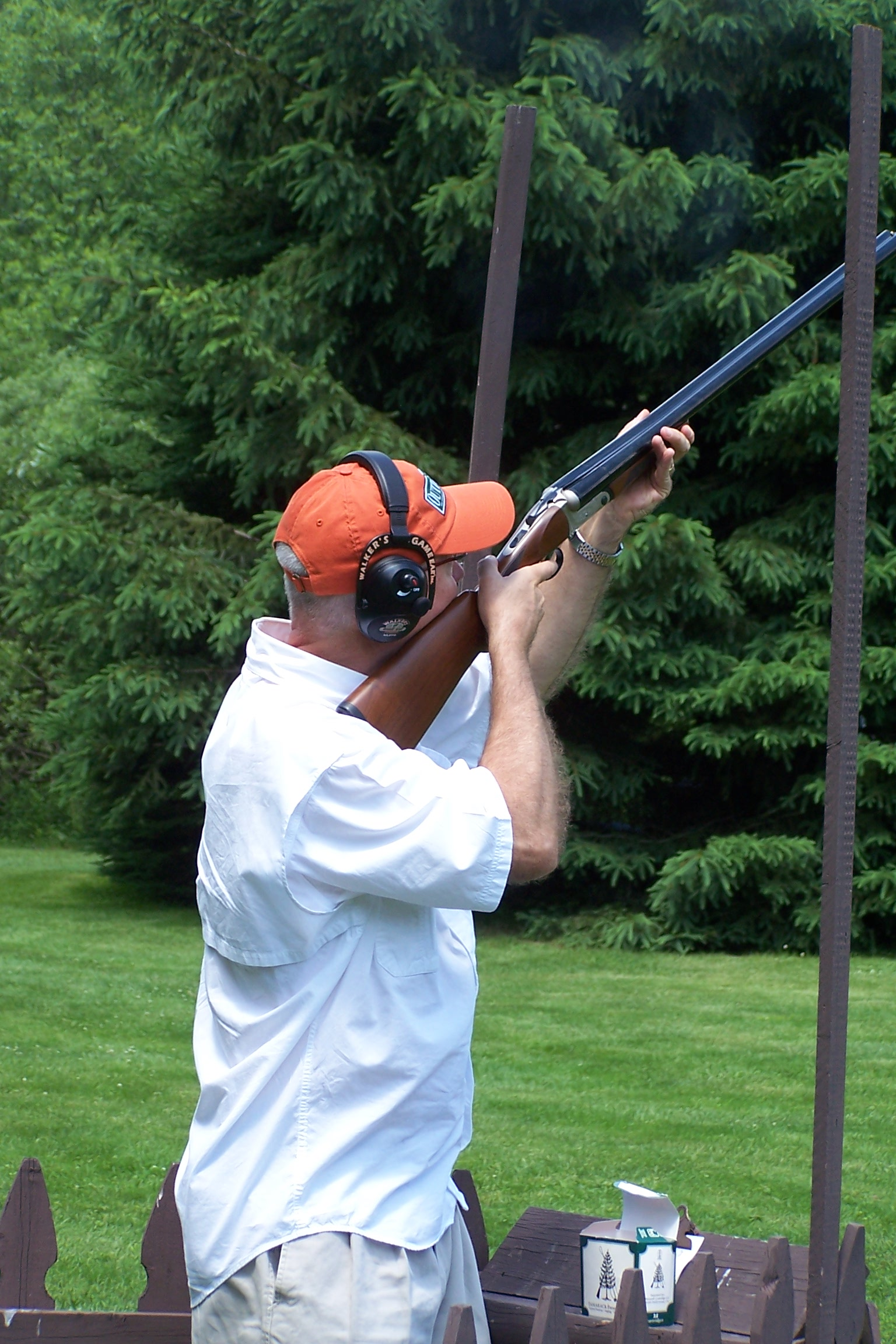SHAREBuck Attacks Hunter

As I said, it is simple arithmetic and the formula for recoil energy is simply E=1/2 MV Squared, with “M” being mass of the gun and “V” being the recoil velocity. In other words, you simply square the velocity of recoil, which is easily calculated when we know the weight of the bullet and powder charge and its velocity when exiting from the muzzle. Multiply by the weight of the gun and divide by twice the acceleration of gravity, and you get the recoil energy in foot pounds. What could be simpler?

Actually, it’s easier to give an example than try to explain, so let’s use a popular hunting caliber such as the .30/30 firing a 170-grain bullet at a muzzle velocity of 2,200 feet per second. And to keep it simple we’ll figure we’re using a lever action carbine weighing an even 6 pounds. And to make it interesting we’ll say the powder charge weighs 30 grains. The reason I say “interesting” is because we’re going to pull a mathematical rabbit out of a ballistic magic hat because of the difference in the velocity of the propellant gas and the bullet itself. We do this by multiplying the powder charge by 1.75, which gives us 52.5 (have your pocket calculator handy, or do you want to do this in your head?). Now we add to this the weight of the bullet, which gives us 222.5. Wow, this is really getting complicated. Let’s take a break.

Now we’re getting to some important numbers by multiplying 222.5 by the muzzle velocity, which gets us 489,500. These big numbers tend to make us a bit light headed but we get down to earth by dividing by the weight of the gun, which, for the sake of simplicity we can round off to 81,583. Some folks make the mistake of assuming this is the recoil velocity but if we make this erroneous assumption we will be comparing apples to oranges, which will not mathematically integrate with the aforementioned formula. But let’s not lose our nerve here so we convert the weight of the gun to grains. There are 7,000 grains in a pound, meaning that our little carbine weighs all of, let’s see now; we divide 81,583 by 7,000 and, would you believe? We have an honest to goodness recoil velocity of 11.6547, or thereabouts. So now we’re back to the using the simple formula to get recoil energy.

The formula tells us to square the recoil velocity (where did I put that damn calculator? It was here on my desk just a moment ago. Here it is.) and the square is 135.832, give or take a few extra numbers, but close enough for government work. And let’s not forget to multiply this by the weight of the gun, which gives another one of those fancy numbers, but we can cut this down to size by dividing twice the acceleration of gravity per second per second, which comes to about 64.4, which (wonder of wonders!), comes out to 12.655. Well, I’ll be damned! Does this mean the recoil of a little 6 pound .30/30 is 12.655 pounds? Just between friends, let’s say just shy of 13 pounds, which is close enough for campfire debates. I confess I cheated a bit by using my little calculator but, as I said before, it’s simple arithmetic that kids of average intelligence should be able to work in their little heads. Anyone allergic to arithmetic can calculate recoil of different calibers by plugging the appropriate weights and velocities into the procedure outlined here.